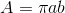# Ellipse Area and Perimeter Calculator

Online calculator to calculate the area A and the circumference C of an ellipse, given by the formulawhereandandare the semi axes of the elipse.

Note that the circumference is given by an infinite sum (series) and therefore cannot be calculated exactly. But taking a large number N of terms in the series, will ensure that the circumference is obtained with a good accuracy. You have the possibility of changing N in the calculator below and see how C converges towards a "fixed value" as you increase N.

## How to use the calculator

Enter the semi axes(0 or greater) and(0 or greater) and N (integer, 1 or greater) the number of terms in the series and press "calculate".== N = A = C =